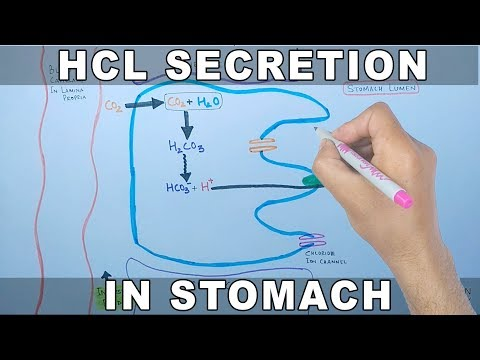Courses

# HCL Technical Paper 1

## 10 Questions MCQ Test Placement Papers - Technical & HR Questions | HCL Technical Paper 1

Description
This mock test of HCL Technical Paper 1 for Quant helps you for every Quant entrance exam. This contains 10 Multiple Choice Questions for Quant HCL Technical Paper 1 (mcq) to study with solutions a complete question bank. The solved questions answers in this HCL Technical Paper 1 quiz give you a good mix of easy questions and tough questions. Quant students definitely take this HCL Technical Paper 1 exercise for a better result in the exam. You can find other HCL Technical Paper 1 extra questions, long questions & short questions for Quant on EduRev as well by searching above.
QUESTION: 1

Solution:
QUESTION: 2

Solution:
QUESTION: 3

### For 1 MB memory number of address lines required,

Solution:
QUESTION: 4

Semaphore is used for

Solution:
QUESTION: 5

class c: public A, public B

Solution:
QUESTION: 6

preproconia.. does not do one of the following

Solution:
*Answer can only contain numeric values
QUESTION: 7

What is the output of the following program?

Y=10; if( Y++>9 && Y++!=10 && Y++>10) printf("........ Y); else printf("".... )

Solution:
QUESTION: 8

What is the output of the following program?

f=(x>y)?x:y

Solution:
QUESTION: 9

If x is even, then (x%2)=0 x &1 !=1 > x! ( some stuff is there)

Solution:
*Multiple options can be correct
QUESTION: 10

Which of the function operator cannot be over loaded

Solution:

Track your progress, build streaks, highlight & save important lessons and more!

### Similar Content### Related tests